Notebook

# Generalized Method of Moments with ARCH and GARCH Models¶

By Delaney Granizo-Mackenzie and Andrei Kirilenko developed as part of the Masters of Finance curriculum at MIT Sloan.

Part of the Quantopian Lecture Series:

Notebook released under the Creative Commons Attribution 4.0 License.

AutoRegressive Conditionally Heteroskedastic (ARCH) occurs when the volatility of a time series is also autoregressive.

In :
import cvxopt
from functools import partial
import math
import numpy as np
import scipy
from scipy import stats
import statsmodels.api as sm
from statsmodels.stats.stattools import jarque_bera

import matplotlib.pyplot as plt


## Simulating a GARCH(1, 1) Case¶

We'll start by using Monte Carlo sampling to simulate a GARCH(1, 1) process. Our dynamics will be

$$\sigma*1 = \sqrt{\frac{a_0}{1-a_1-b_1}}$$$$\sigma_t^2 = a_0 + a_1 x*{t-1}^2+b*1 \sigma*{t-1}^2$$$$x_t = \sigma_t \epsilon_t$$$$\epsilon \sim \mathcal{N}(0, 1)$$

Our parameters will be $a_0 = 1$, $a_1=0.1$, and $b_1=0.8$. We will drop the first 10% (burn-in) of our simulated values.

In :
# Define parameters
a0 = 1.0
a1 = 0.1
b1 = 0.8
sigma1 = math.sqrt(a0 / (1 - a1 - b1))

In :
def simulate_GARCH(T, a0, a1, b1, sigma1):

# Initialize our values
X = np.ndarray(T)
sigma = np.ndarray(T)
sigma = sigma1

for t in range(1, T):
# Draw the next x_t
X[t - 1] = sigma[t - 1] * np.random.normal(0, 1)
# Draw the next sigma_t
sigma[t] = math.sqrt(a0 + b1 * sigma[t - 1]**2 + a1 * X[t - 1]**2)

X[T - 1] = sigma[T - 1] * np.random.normal(0, 1)

return X, sigma


Now we'll compare the tails of the GARCH(1, 1) process with normally distributed values. We expect to see fatter tails, as the GARCH(1, 1) process will experience extreme values more often.

In :
X, _ = simulate_GARCH(10000, a0, a1, b1, sigma1)
X = X[1000:] # Drop burn in
X = X / np.std(X) # Normalize X

def compare_tails_to_normal(X):
# Define matrix to store comparisons
A = np.zeros((2,4))
for k in range(4):
A[0, k] = len(X[X > (k + 1)]) / float(len(X)) # Estimate tails of X
A[1, k] = 1 - stats.norm.cdf(k + 1) # Compare to Gaussian distribution
return A

compare_tails_to_normal(X)

Out:
array([[  1.54888889e-01,   2.41111111e-02,   1.11111111e-03,
0.00000000e+00],
[  1.58655254e-01,   2.27501319e-02,   1.34989803e-03,
3.16712418e-05]])

Sure enough, the tails of the GARCH(1, 1) process are fatter. We can also look at this graphically, although it's a little tricky to see.

In :
plt.hist(X, bins=50)
plt.xlabel('sigma')
plt.ylabel('observations');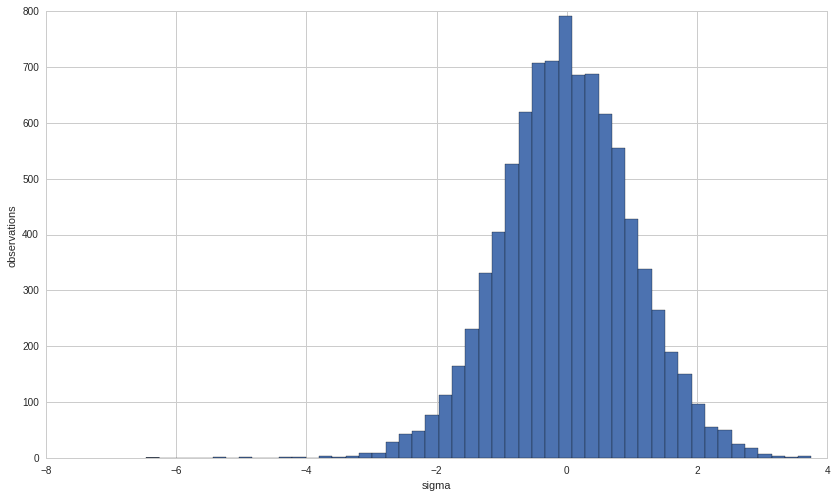In :
# Sample values from a normal distribution
X2 = np.random.normal(0, 1, 9000)
both = np.matrix([X, X2])

In :
# Plot both the GARCH and normal values
plt.plot(both.T, alpha=.7);
plt.axhline(X2.std(), color='yellow', linestyle='--')
plt.axhline(-X2.std(), color='yellow', linestyle='--')
plt.axhline(3*X2.std(), color='red', linestyle='--')
plt.axhline(-3*X2.std(), color='red', linestyle='--')
plt.xlabel('time')
plt.ylabel('sigma');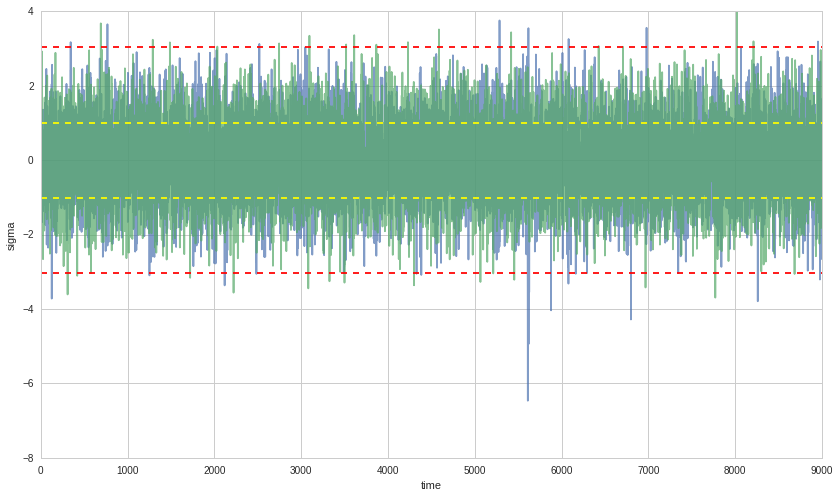What we're looking at here is the GARCH process in blue and the normal process in green. The 1 and 3 std bars are drawn on the plot. We can see that the blue GARCH process tends to cross the 3 std bar much more often than the green normal one.

## Testing for ARCH Behavior¶

The first step is to test for ARCH conditions. To do this we run a regression on $x_t$ fitting the following model.

$$x*t^2 = a_0 + a_1 x*{t-1}^2 + \dots + a*p x*{t-p}^2$$

We use OLS to estimate $\hat\theta = (\hat a_0, \hat a_1, \dots, \hat a_p)$ and the covariance matrix $\hat\Omega$. We can then compute the test statistic

$$F = \hat\theta \hat\Omega^{-1} \hat\theta'$$

We will reject if $F$ is greater than the 95% confidence bars in the $\mathcal(X)^2(p)$ distribution.

To test, we'll set $p=20$ and see what we get.

In :
X, _ = simulate_GARCH(1100, a0, a1, b1, sigma1)
X = X[100:] # Drop burn in

p = 20

# Drop the first 20 so we have a lag of p's
Y2 = (X**2)[p:]
X2 = np.ndarray((980, p))
for i in range(p, 1000):
X2[i - p, :] = np.asarray((X**2)[i-p:i])[::-1]

model = sm.OLS(Y2, X2)
model = model.fit()
theta = np.matrix(model.params)
omega = np.matrix(model.cov_HC0)
F = np.asscalar(theta * np.linalg.inv(omega) * theta.T)

print np.asarray(theta.T).shape

plt.plot(range(20), np.asarray(theta.T))
plt.xlabel('Lag Amount')
plt.ylabel('Estimated Coefficient for Lagged Datapoint')

print 'F = ' + str(F)

chi2dist = scipy.stats.chi2(p)
pvalue = 1-chi2dist.cdf(F)
print 'p-value = ' + str(pvalue)

# Finally let's look at the significance of each a_p as measured by the standard deviations away from 0
print theta/np.diag(omega)

(20, 1)
F = 341.736612805
p-value = 0.0
[[ 82.59140112  71.46296619  42.80265659   5.9693111    5.61141539
30.89781715  36.54379448  21.58236845  30.66660757 -10.92922828
13.41504385  48.73313057  10.17361295 -70.71538298  23.39274787
40.53162163  56.12994402 -28.95568019  42.05335583  35.05024461]]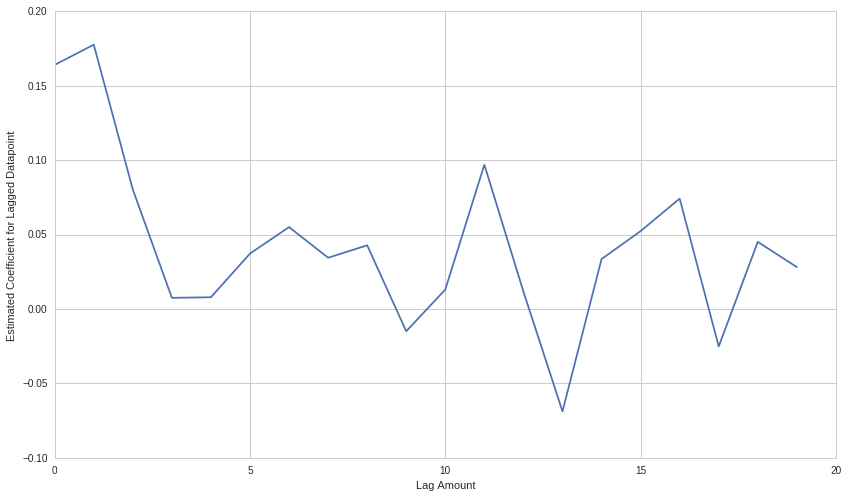## Fitting GARCH(1, 1) with MLE¶

Once we've decided that the data might have an underlying GARCH(1, 1) model, we would like to fit GARCH(1, 1) to the data by estimating parameters.

To do this we need the log-likelihood function

$$\mathcal{L}(\theta) = \sum_{t=1}^T - \ln \sqrt{2\pi} - \frac{x_t^2}{2\sigma_t^2} - \frac{1}{2}\ln(\sigma_t^2)$$

To evaluate this function we need $x_t$ and $\sigma_t$ for $1 \leq t \leq T$. We have $x_t$, but we need to compute $\sigma_t$. To do this we need to make a guess for $\sigma_1$. Our guess will be $\sigma_1^2 = \hat E[x_t^2]$. Once we have our initial guess we compute the rest of the $\sigma$'s using the equation

$$\sigma*t^2 = a_0 + a_1 x*{t-1}^2 + b*1\sigma*{t-1}^2$$
In :
X, _ = simulate_GARCH(10000, a0, a1, b1, sigma1)
X = X[1000:] # Drop burn in

In :
# Here's our function to compute the sigmas given the initial guess
def compute_squared_sigmas(X, initial_sigma, theta):

a0 = theta
a1 = theta
b1 = theta

T = len(X)
sigma2 = np.ndarray(T)

sigma2 = initial_sigma ** 2

for t in range(1, T):
# Here's where we apply the equation
sigma2[t] = a0 + a1 * X[t-1]**2 + b1 * sigma2[t-1]

return sigma2


Let's look at the sigmas we just generated.

In :
plt.plot(range(len(X)), compute_squared_sigmas(X, np.sqrt(np.mean(X**2)), (1, 0.5, 0.5)))
plt.xlabel('Time')
plt.ylabel('Sigma');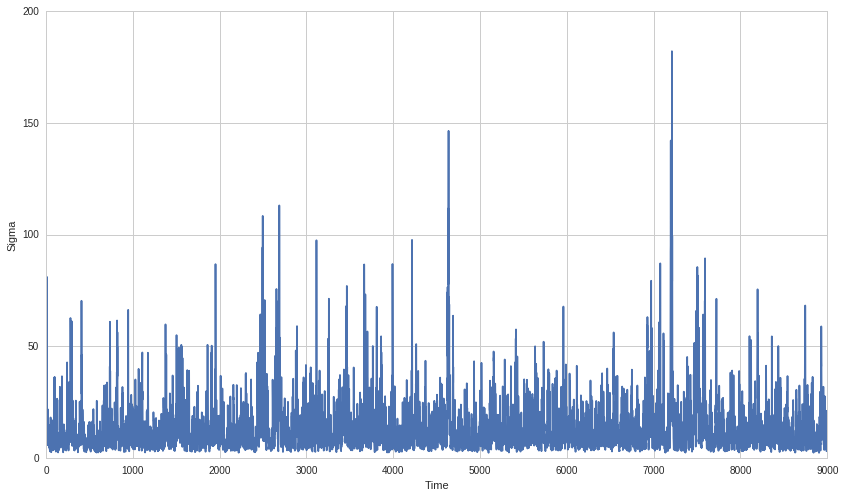Now that we can compute the $\sigma_t$'s, we'll define the actual log likelihood function. This function will take as input our observations $x$ and $\theta$ and return $-\mathcal{L}(\theta)$. It is important to note that we return the negative log likelihood, as this way our numerical optimizer can minimize the function while maximizing the log likelihood.

Note that we are constantly re-computing the $\sigma_t$'s in this function.

In :
def negative_log_likelihood(X, theta):

T = len(X)

# Estimate initial sigma squared
initial_sigma = np.sqrt(np.mean(X ** 2))

# Generate the squared sigma values
sigma2 = compute_squared_sigmas(X, initial_sigma, theta)

# Now actually compute
return -sum(
[-np.log(np.sqrt(2.0 * np.pi)) -
(X[t] ** 2) / (2.0 * sigma2[t]) -
0.5 * np.log(sigma2[t]) for
t in range(T)]
)


Now we perform numerical optimization to find our estimate for $$\hat\theta = \arg \max*{(a_0, a_1, b_1)}\mathcal{L}(\theta) = \arg \min*{(a_0, a_1, b_1)}-\mathcal{L}(\theta)$$

We have some constraints on this

$$a_1 \geq 0, b_1 \geq 0, a_1+b_1 < 1$$
In :
# Make our objective function by plugging X into our log likelihood function
objective = partial(negative_log_likelihood, X)

# Define the constraints for our minimizer
def constraint1(theta):
return np.array([1 - (theta + theta)])

def constraint2(theta):
return np.array([theta])

def constraint3(theta):
return np.array([theta])

cons = ({'type': 'ineq', 'fun': constraint1},
{'type': 'ineq', 'fun': constraint2},
{'type': 'ineq', 'fun': constraint3})

# Actually do the minimization
result = scipy.optimize.minimize(objective, (1, 0.5, 0.5),
method='SLSQP',
constraints = cons)
theta_mle = result.x
print 'theta MLE: ' + str(theta_mle)

theta MLE: [ 0.89882685  0.10272342  0.81126578]


Now we would like a way to check our estimate. We'll look at two things:

1. How fat are the tails of the residuals.
2. How normal are the residuals under the Jarque-Bera normality test.

We'll do both in our check_theta_estimate function.

In :
def check_theta_estimate(X, theta_estimate):
initial_sigma = np.sqrt(np.mean(X ** 2))
sigma = np.sqrt(compute_squared_sigmas(X, initial_sigma, theta_estimate))
epsilon = X / sigma
print 'Tails table'
print compare_tails_to_normal(epsilon / np.std(epsilon))
print ''

_, pvalue, _, _ = jarque_bera(epsilon)
print 'Jarque-Bera probability normal: ' + str(pvalue)

check_theta_estimate(X, theta_mle)

Tails table
[[  1.58888889e-01   2.31111111e-02   1.22222222e-03   0.00000000e+00]
[  1.58655254e-01   2.27501319e-02   1.34989803e-03   3.16712418e-05]]

Jarque-Bera probability normal: 0.547561671115


## GMM for Estimating GARCH(1, 1) Parameters¶

We've just computed an estimate using MLE, but we can also use Generalized Method of Moments (GMM) to estimate the GARCH(1, 1) parameters.

To do this we need to define our moments. We'll use 4.

1. The residual $\hat\epsilon_t = x_t / \hat\sigma_t$
2. The variance of the residual $\hat\epsilon_t^2$
3. The skew moment $\mu_3/\hat\sigma_t^3 = (\hat\epsilon_t - E[\hat\epsilon_t])^3 / \hat\sigma_t^3$
4. The kurtosis moment $\mu_4/\hat\sigma_t^4 = (\hat\epsilon_t - E[\hat\epsilon_t])^4 / \hat\sigma_t^4$
In :
# The n-th standardized moment
# skewness is 3, kurtosis is 4
def standardized_moment(x, mu, sigma, n):
return ((x - mu) ** n) / (sigma ** n)


GMM now has three steps.

Start with $W$ as the identity matrix.

1. Estimate $\hat\theta*1$ by using numerical optimization to minimize $$\min*{\theta \in \Theta} \left(\frac{1}{T} \sum*{t=1}^T g(x_t, \hat\theta)\right)' W \left(\frac{1}{T}\sum*{t=1}^T g(x_t, \hat\theta)\right)$$
2. Recompute $W$ based on the covariances of the estimated $\theta$. (Focus more on parameters with explanatory power) $$\hat W*{i+1} = \left(\frac{1}{T}\sum*{t=1}^T g(x_t, \hat\theta_i)g(x_t, \hat\theta_i)'\right)^{-1}$$
3. Repeat until $|\hat\theta_{i+1} - \hat\theta_i| < \epsilon$ or we reach an iteration threshold.

Initialize $W$ and $T$ and define the objective function we need to minimize.

In :
def gmm_objective(X, W, theta):
# Compute the residuals for X and theta
initial_sigma = np.sqrt(np.mean(X ** 2))
sigma = np.sqrt(compute_squared_sigmas(X, initial_sigma, theta))
e = X / sigma

# Compute the mean moments
m1 = np.mean(e)
m2 = np.mean(e ** 2) - 1
m3 = np.mean(standardized_moment(e, np.mean(e), np.std(e), 3))
m4 = np.mean(standardized_moment(e, np.mean(e), np.std(e), 4) - 3)

G = np.matrix([m1, m2, m3, m4]).T

return np.asscalar(G.T * W * G)

def gmm_variance(X, theta):
# Compute the residuals for X and theta
initial_sigma = np.sqrt(np.mean(X ** 2))
sigma = np.sqrt(compute_squared_sigmas(X, initial_sigma, theta))
e = X / sigma

# Compute the squared moments
m1 = e ** 2
m2 = (e ** 2 - 1) ** 2
m3 = standardized_moment(e, np.mean(e), np.std(e), 3) ** 2
m4 = (standardized_moment(e, np.mean(e), np.std(e), 4) - 3) ** 2

# Compute the covariance matrix g * g'
T = len(X)
s = np.ndarray((4, 1))
for t in range(T):
G = np.matrix([m1[t], m2[t], m3[t], m4[t]]).T
s = s + G * G.T

return s / T


Now we're ready to the do the iterated minimization step.

In :
# Initialize GMM parameters
W = np.identity(4)
gmm_iterations = 10

# First guess
theta_gmm_estimate = theta_mle

# Perform iterated GMM
for i in range(gmm_iterations):
# Estimate new theta
objective = partial(gmm_objective, X, W)
result = scipy.optimize.minimize(objective, theta_gmm_estimate, constraints=cons)
theta_gmm_estimate = result.x
print 'Iteration ' + str(i) + ' theta: ' + str(theta_gmm_estimate)

# Recompute W
W = np.linalg.inv(gmm_variance(X, theta_gmm_estimate))

check_theta_estimate(X, theta_gmm_estimate)

Iteration 0 theta: [ 0.898825    0.10283861  0.81129305]
Iteration 1 theta: [ 0.89881434  0.1027472   0.81118925]
Iteration 2 theta: [ 0.89881434  0.1027472   0.81118925]
Iteration 3 theta: [ 0.89881434  0.1027472   0.81118925]
Iteration 4 theta: [ 0.89881434  0.1027472   0.81118925]
Iteration 5 theta: [ 0.89881434  0.1027472   0.81118925]
Iteration 6 theta: [ 0.89881434  0.1027472   0.81118925]
Iteration 7 theta: [ 0.89881434  0.1027472   0.81118925]
Iteration 8 theta: [ 0.89881434  0.1027472   0.81118925]
Iteration 9 theta: [ 0.89881434  0.1027472   0.81118925]
Tails table
[[  1.58888889e-01   2.32222222e-02   1.22222222e-03   0.00000000e+00]
[  1.58655254e-01   2.27501319e-02   1.34989803e-03   3.16712418e-05]]

Jarque-Bera probability normal: 0.547586182431


## Predicting the Future: How to actually use what we've done¶

Now that we've fitted a model to our observations, we'd like to be able to predict what the future volatility will look like. To do this, we can just simulate more values using our original GARCH dynamics and the estimated parameters.

The first thing we'll do is compute an initial $\sigma_t$. We'll compute our squared sigmas and take the last one.

In :
sigma_hats = np.sqrt(compute_squared_sigmas(X, np.sqrt(np.mean(X**2)), theta_mle))
initial_sigma = sigma_hats[-1]
initial_sigma

Out:
2.9977470389171796

Now we'll just sample values walking forward.

In :
a0_estimate = theta_gmm_estimate
a1_estimate = theta_gmm_estimate
b1_estimate = theta_gmm_estimate

X_forecast, sigma_forecast = simulate_GARCH(100, a0_estimate, a1_estimate, b1_estimate, initial_sigma)

In :
plt.plot(range(-100, 0), X[-100:], 'b-')
plt.plot(range(-100, 0), sigma_hats[-100:], 'r-')
plt.plot(range(0, 100), X_forecast, 'b--')
plt.plot(range(0, 100), sigma_forecast, 'r--')
plt.xlabel('Time')
plt.legend(['X', 'sigma']);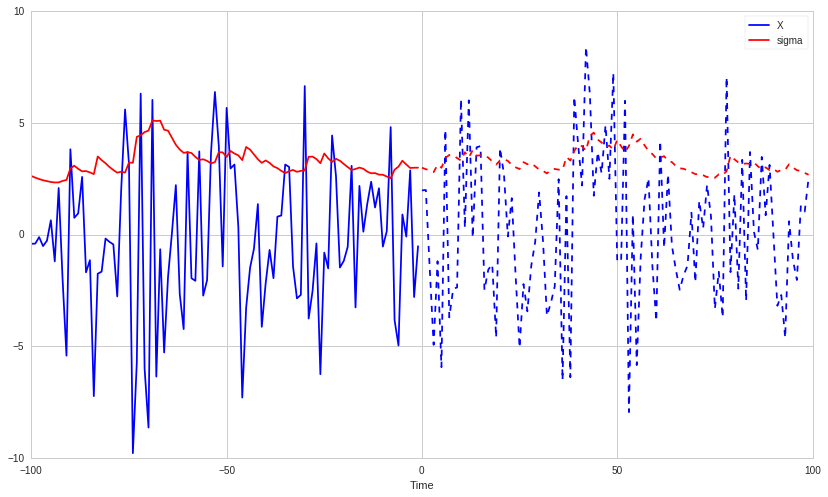One should note that because we are moving foward using a random walk, this analysis is supposed to give us a sense of the magnitude of sigma and therefore the risk we could face. It is not supposed to accurately model future values of X. In practice you would probably want to use Monte Carlo sampling to generate thousands of future scenarios, and then look at the potential range of outputs. We'll try that now. Keep in mind that this is a fairly simplistic way of doing this analysis, and that better techniques, such as Bayesian cones, exist.

In :
plt.plot(range(-100, 0), X[-100:], 'b-')
plt.plot(range(-100, 0), sigma_hats[-100:], 'r-')
plt.xlabel('Time')
plt.legend(['X', 'sigma'])

max_X = [-np.inf]
min_X = [np.inf]
for i in range(100):
X_forecast, sigma_forecast = simulate_GARCH(100, a0_estimate, a1_estimate, b1_estimate, initial_sigma)
if max(X_forecast) > max(max_X):
max_X = X_forecast
elif min(X_forecast) < min(max_X):
min_X = X_forecast
plt.plot(range(0, 100), X_forecast, 'b--', alpha=0.05)
plt.plot(range(0, 100), sigma_forecast, 'r--', alpha=0.05)

# Draw the most extreme X values specially
plt.plot(range(0, 100), max_X, 'g--', alpha=1.0)
plt.plot(range(0, 100), min_X, 'g--', alpha=1.0);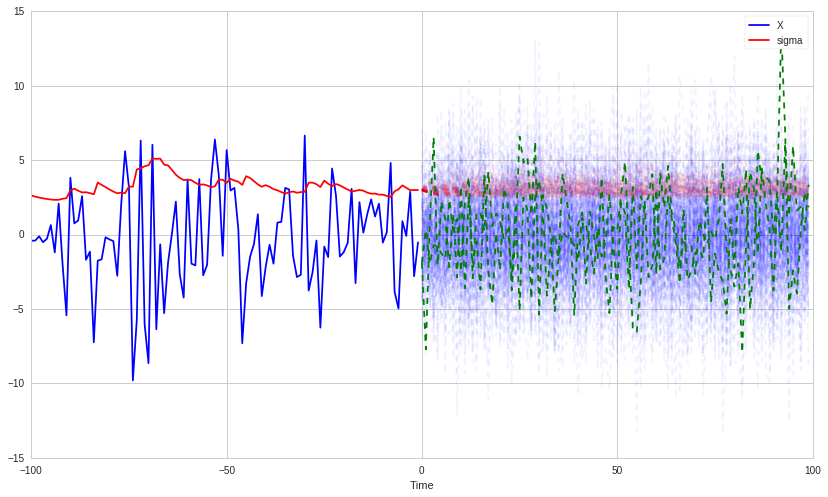This presentation is for informational purposes only and does not constitute an offer to sell, a solicitation to buy, or a recommendation for any security; nor does it constitute an offer to provide investment advisory or other services by Quantopian, Inc. ("Quantopian"). Nothing contained herein constitutes investment advice or offers any opinion with respect to the suitability of any security, and any views expressed herein should not be taken as advice to buy, sell, or hold any security or as an endorsement of any security or company. In preparing the information contained herein, Quantopian, Inc. has not taken into account the investment needs, objectives, and financial circumstances of any particular investor. Any views expressed and data illustrated herein were prepared based upon information, believed to be reliable, available to Quantopian, Inc. at the time of publication. Quantopian makes no guarantees as to their accuracy or completeness. All information is subject to change and may quickly become unreliable for various reasons, including changes in market conditions or economic circumstances.